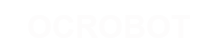#### Site Tools

en:reference:language:bitshift

# Differences

This shows you the differences between two versions of the page.

 — en:reference:language:bitshift [2017/04/07 10:03] (current) 2014/11/13 15:17 弘毅 created 2014/11/13 15:17 弘毅 created Line 1: Line 1: + ====== bitshift left (<<), bitshift right (>>) ====== + + + ===== Description ===== + + From The Bitmath Tutorial in The Playground + + + There are two bit shift operators in C++: the left shift operator << and the right shift operator >>. These operators cause the bits in the left operand to be shifted left or right by the number of positions specified by the right operand. + + + More on bitwise math may be found here. + ===== Syntax ===== + + variable << number_of_bits + variable >> number_of_bits + ===== Parameters ===== + + variable - (byte, int, long) number_of_bits integer <= 32 + ===== Example: ===== + + int a = 5;        // binary: 0000000000000101 + int b = a << 3;   // binary: 0000000000101000,​ or 40 in decimal + int c = b >> 3;   // binary: 0000000000000101,​ or back to 5 like we started with +  ​ + When you shift a value x by y bits (x << y), the leftmost y bits in x are lost, literally shifted out of existence: + + int a = 5;        // binary: 0000000000000101 + int b = a << 14;  // binary: 0100000000000000 - the first 1 in 101 was discarded + ​ + If you are certain that none of the ones in a value are being shifted into oblivion, a simple way to think of the left-shift operator is that it multiplies the left operand by 2 raised to the right operand power. For example, to generate powers of 2, the following expressions can be employed: + + 1 << ​ 0  ==    1 + 1 << ​ 1  ==    2 + 1 << ​ 2  ==    4 + 1 << ​ 3  ==    8 + ... + 1 << ​ 8  ==  256 + 1 << ​ 9  ==  512 + 1 << 10  == 1024 + ... + ​ + When you shift x right by y bits (x >> y), and the highest bit in x is a 1, the behavior depends on the exact data type of x. If x is of type int, the highest bit is the sign bit, determining whether x is negative or not, as we have discussed above. In that case, the sign bit is copied into lower bits, for esoteric historical reasons: + + int x = -16;     // binary: 1111111111110000 + int y = x >> 3;  // binary: 1111111111111110 + ​ + This behavior, called sign extension, is often not the behavior you want. Instead, you may wish zeros to be shifted in from the left. It turns out that the right shift rules are different for unsigned int expressions,​ so you can use a typecast to suppress ones being copied from the left: + + int x = -16;                   // binary: 1111111111110000 + int y = (unsigned int)x >> 3;  // binary: 0001111111111110 + ​ + If you are careful to avoid sign extension, you can use the right-shift operator >> as a way to divide by powers of 2. For example: + + int x = 1000; + int y = x >> 3;   // integer division of 1000 by 8, causing y = 125. +